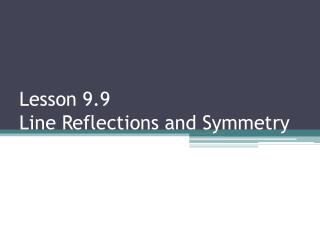DownloadDownload PresentationLesson 9.9 Line Reflections and Symmetry

# Lesson 9.9 Line Reflections and Symmetry

Télécharger la présentation## Lesson 9.9 Line Reflections and Symmetry

- - - - - - - - - - - - - - - - - - - - - - - - - - - E N D - - - - - - - - - - - - - - - - - - - - - - - - - - -
##### Presentation Transcript

1. Lesson 9.9 Line Reflections and Symmetry

2. Line of Symmetry • Divides the figure in two congruent halves.

3. Line Reflections y A C B x Line Reflection - Reflection occurs when points are redrawn across a line of symmetry at equal distance. Coordinate Notation “Opposite of” x y -x y -5 4 5 4 -5 1 5 1 -1 1 1 1 Reflect over Y-AXIS (-x, y) – opposite of x Reflect over X-AXIS (x, -y) – opposite of y

4. y x Points L(-3,2), M(3,1), and N(-2,-2) are the vertices of a triangle. If LMN is reflected over the x-axis, what are the coordinates of its image? Coordinate Notation L M N

5. Homework Page 487 (8-19) all